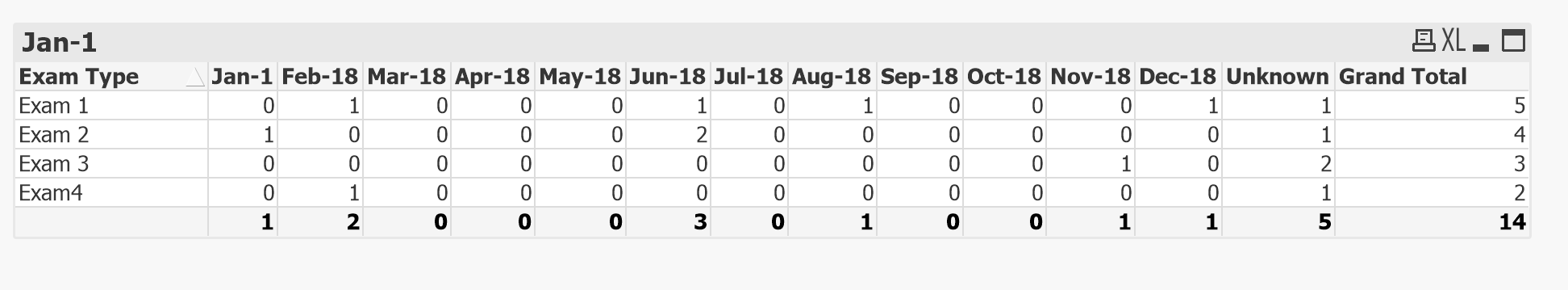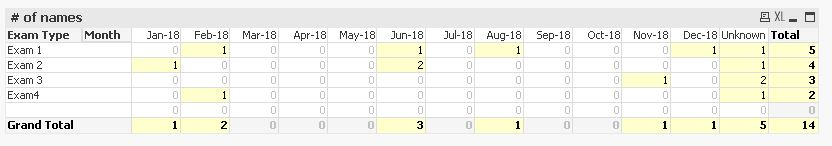# QlikView App Development

Discussion Board for collaboration related to QlikView App Development.

Announcements
Leverage your QlikView investment to modernize BI – see how! Join Group
cancel
Showing results for
Did you mean:
HighlightedContributor III

## Values in a Table become a Column

Hi Qlikers,

I have the following underlying data table in excel

NameExam 1Exam 2Exam 3Exam4
SteveJun-18Jun-18Nov-18Unknown
LouiseFeb-18Jun-18
MichaelUnknownUnknownUnknown
JoeAug-18Feb-18

I need to transpose this into Qlikview and create the table below. [i.e. the date and text values in the above table become columns, and the values of the below are a count of the Names]

Exam TypeJan-18Feb-18Mar-18Apr-18May-18Jun-18Jul-18Aug-18Sep-18Oct-18Nov-18Dec-18UnknownGrand Total
Exam 1111115
Exam 21214
Exam 3123
Exam 4112
Grand Total120003010011514

Is this possible, I have read some other forums and it seems Cross Tables may be the solution, but have gone through them I still can't make it work. Is any able to demonstrate this please.

Many Thanks

Kozan

5 Replies
HighlightedMaster III

## Re: Values in a Table become a Column

Try like this -

Table1:

CrossTable([Exam Type], Data)

[Exam 1],

[Exam 2],

[Exam 3],

Exam4

FROM

(html, codepage is 1252, embedded labels, table is @1);

Table2:

[Exam Type],

Count(If (([Exam Type]='Exam 1' or [Exam Type]='Exam 2' or [Exam Type]='Exam 3' or [Exam Type]='Exam 4') and Data='Jan-18',Data)) as [Jan-18],

Count(If (([Exam Type]='Exam 1' or [Exam Type]='Exam 2' or [Exam Type]='Exam 3' or [Exam Type]='Exam 4') and Data='Feb-18',Data)) as [Feb-18],

Count(If (([Exam Type]='Exam 1' or [Exam Type]='Exam 2' or [Exam Type]='Exam 3' or [Exam Type]='Exam 4') and Data='Mar-18',Data)) as [Mar-18],

Count(If (([Exam Type]='Exam 1' or [Exam Type]='Exam 2' or [Exam Type]='Exam 3' or [Exam Type]='Exam 4') and Data='Apr-18',Data)) as [Apr-18],

Count(If (([Exam Type]='Exam 1' or [Exam Type]='Exam 2' or [Exam Type]='Exam 3' or [Exam Type]='Exam 4') and Data='May-18',Data)) as [May-18],

Count(If (([Exam Type]='Exam 1' or [Exam Type]='Exam 2' or [Exam Type]='Exam 3' or [Exam Type]='Exam 4') and Data='Jun-18',Data)) as [Jun-18],

Count(If (([Exam Type]='Exam 1' or [Exam Type]='Exam 2' or [Exam Type]='Exam 3' or [Exam Type]='Exam 4') and Data='Jul-18',Data)) as [Jul-18],

Count(If (([Exam Type]='Exam 1' or [Exam Type]='Exam 2' or [Exam Type]='Exam 3' or [Exam Type]='Exam 4') and Data='Aug-18',Data)) as [Aug-18],

Count(If (([Exam Type]='Exam 1' or [Exam Type]='Exam 2' or [Exam Type]='Exam 3' or [Exam Type]='Exam 4') and Data='Sep-18',Data)) as [Sep-18],

Count(If (([Exam Type]='Exam 1' or [Exam Type]='Exam 2' or [Exam Type]='Exam 3' or [Exam Type]='Exam 4') and Data='Oct-18',Data)) as [Oct-18],

Count(If (([Exam Type]='Exam 1' or [Exam Type]='Exam 2' or [Exam Type]='Exam 3' or [Exam Type]='Exam 4') and Data='Nov-18',Data)) as [Nov-18],

Count(If (([Exam Type]='Exam 1' or [Exam Type]='Exam 2' or [Exam Type]='Exam 3' or [Exam Type]='Exam 4') and Data='Dec-18',Data)) as [Dec-18],

Count(If (([Exam Type]='Exam 1' or [Exam Type]='Exam 2' or [Exam Type]='Exam 3' or [Exam Type]='Exam 4') and Data='Unknown',Data)) as [Unknown]

Resident Table1

Group By [Exam Type];

Drop table Table1;

HighlightedMaster III

## Re: Values in a Table become a ColumnHighlightedMVP

## Re: Values in a Table become a Column

Hi,

maybe one solution could be:```tabTemp:
CrossTable ([Exam Type],MonthTemp)
LOAD * FROM [https://community.qlik.com/thread/294611] (html, codepage is 1252, embedded labels, table is @1);

tabExams:
[Exam Type],
Date#(MonthTemp,'MMM-YY') as Month
Resident tabTemp
Where Len(MonthTemp);

DROP Table tabTemp;

tabMonths:
While MonthName(MinMonth,IterNo()-1) <= MaxMonth;
Max(Month) as MaxMonth
Resident tabExams;
```

hope this helps

regards

Marco

HighlightedContributor III

## Re: Values in a Table become a Column

Thanks Marco.

But it doesn't seem to be working for me.

Issue 1. My Months come out in number format [i.e 42736,42767 etc.] not MMM-YY as desired,

Issue 2: The count doesn't seem to work. I receive the same number across all months. e.g. my database has 3000 entries, this value appear everywhere under each Exam Type.

Unfortunately, I cannot share what I am working on as this is for work. I hope the above makes sense.

Many Thanks

Kozan

HighlightedMVP

Hi,